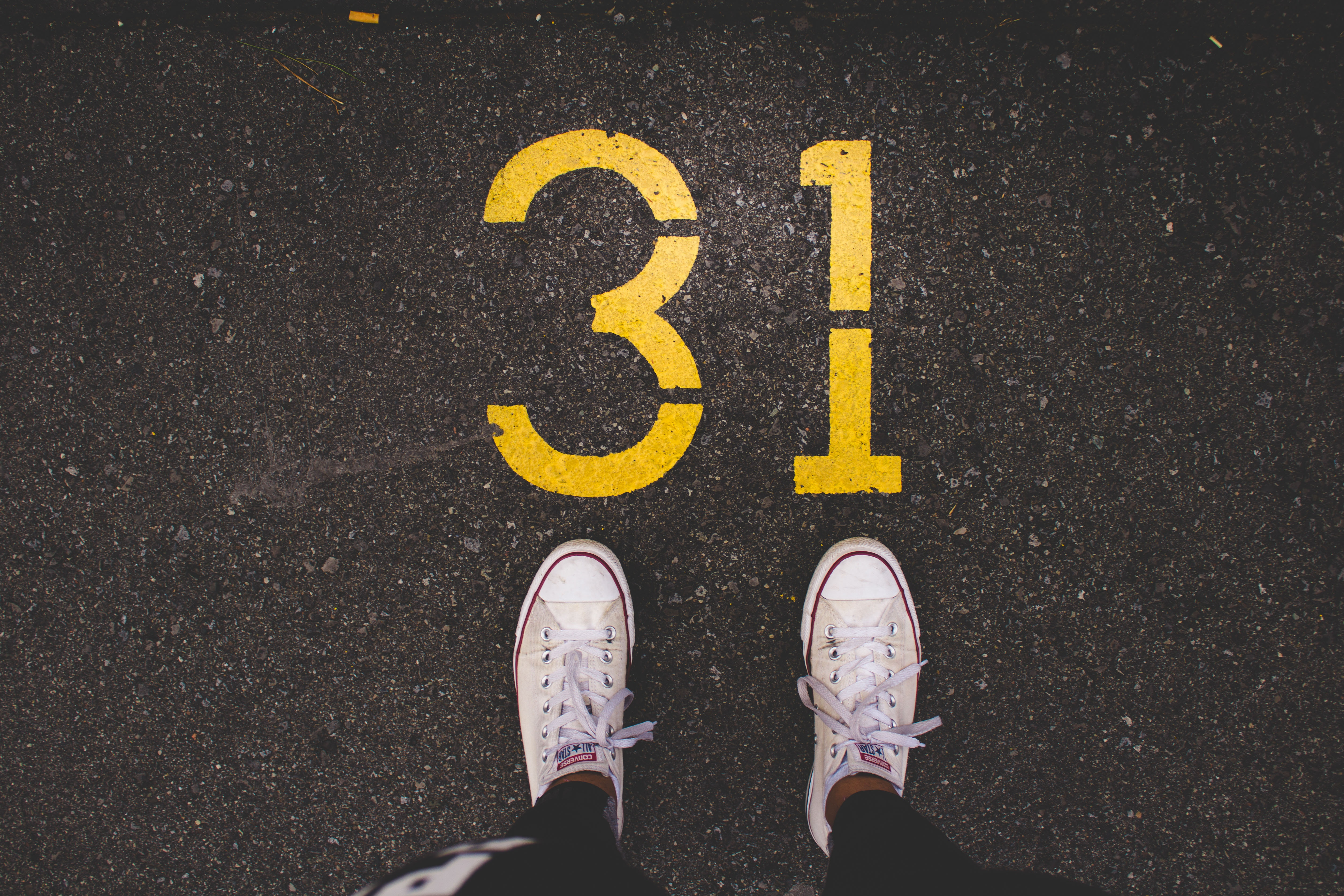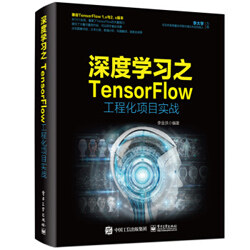# TensorFlow 工程实战（二）：用 tf.layers API 在动态图上识别手写数字## 实例描述

### 一、启动动态图并加载手写图片数据集

import tensorflow as tfimport tensorflow.contrib.eager as tfetf.enable_eager_execution()print("TensorFlow 版本: {}".format(tf.VERSION))import tensorflow_datasets as tfdsimport numpy as np#加载训练和验证数据集ds_train, ds_test = tfds.load(name="mnist", split=["train", "test"])ds_train = ds_train.shuffle(1000).batch(10).prefetch(tf.data.experimental.AUTOTUNE)

### 二、定义模型的类

• __init__ 用于定义网络的各个操作层。本实例中所用到的卷积网络、全连接网络都是用 tf.layers 实现的，其用法与 TF-slim 接口非常相似。
• call 用于将网络中的各层链接起来，形成正向运算的神经网络。

dropout 是一种改善过拟合的方法。通过随机丢弃部分网络节点来忽略数据集中的小概率样本。

class MNISTModel(tf.layers.Layer):							#定义模型类  def __init__(self, name):    super(MNISTModel, self).__init__(name=name)     self._input_shape = [-1, 28, 28, 1]   					#定义输入形状    #定义卷积层    self.conv1 =tf.layers.Conv2D(32, 5,  activation=tf.nn.relu)    #定义卷积层    self.conv2 = tf.layers.Conv2D(64, 5,  activation=tf.nn.relu)    #定义全连接层    self.fc1 =tf.layers.Dense(1024, activation=tf.nn.relu)    self.fc2 = tf.layers.Dense(10)    self.dropout = tf.layers.Dropout(0.5) #定义 dropout 层    #定义池化层    self.max_pool2d = tf.layers.MaxPooling2D(            (2, 2), (2, 2), padding='SAME')   def call(self, inputs, training):						#定义 call 方法    x = tf.reshape(inputs, self._input_shape)				#将网络连接起来    x = self.conv1(x)    x = self.max_pool2d(x)    x = self.conv2(x)    x = self.max_pool2d(x)    x = tf.keras.layers.Flatten()(x)    x = self.fc1(x)    if training:      x = self.dropout(x)    x = self.fc2(x)    return x

### 三、定义网络的反向传播

def loss(model,inputs, labels):    predictions = model(inputs, training=True)        cost = tf.nn.sparse_softmax_cross_entropy_with_logits( logits=predictions, labels=labels )    return tf.reduce_mean( cost )#训练optimizer = tf.train.AdamOptimizer(learning_rate=1e-4)grad = tfe.implicit_gradients(loss)

### 四、训练模型

model = MNISTModel("net")     			#实例化模型global_step = tf.train.get_or_create_global_step()for epoch in range(1):					#按照指定次数迭代数据集    for i,data  in enumerate (ds_train):          			        inputs, targets = tf.cast( data["image"],tf.float32), data["label"]        optimizer.apply_gradients(grad( model,inputs, targets) , global_step=global_step)        if i % 100 == 0:          print("Step %d: Loss on training set : %f" %            (i, loss(model,inputs, targets).numpy()))          #获取要保存的变量          all_variables = ( model.variables + optimizer.variables() + [global_step])          tfe.Saver(all_variables).save(  	#生成检查点文件          "./tfelog/linermodel.cpkt", global_step=global_step)ds = tfds.as_numpy(ds_test.batch(100))onetestdata = next(ds)print("Loss on test set: %f" % loss( model,onetestdata["image"].astype(np.float32), onetestdata["label"]).numpy())

TensorFlow 版本: 1.13.1
Step 0: Loss on training set : 2.252767
……
Step 5600: Loss on training set : 0.002125
Loss on test set: 0.055677TensorFlow 工程实战（一）：用 TF-Hub 库微调模型评估人物年龄
TensorFlow 工程实战（二）：用 tf.layers API 在动态图上识别手写数字（本文）
TensorFlow 工程实战（三）：结合知识图谱实现电影推荐系统
TensorFlow 工程实战（四）：使用带注意力机制的模型分析评论者是否满意
TensorFlow 工程实战（五）：构建 DeblurGAN 模型，将模糊相片变清晰
TensorFlow 工程实战（六）：在 iPhone 手机上识别男女并进行活体检测